Astroparticle Physics
Volume 27, Issue 1, February 2007, Pages 92-98

Superluminal γ-rays from the Crab pulsar

aDepartment of Physics, Hiroshima University, 1-3-1 Kagami-yama, Higashi-Hiroshima 739-8526, Japan

revised 8 August 2006;
accepted 12 September 2006.
Available online 5 October 2006.

### Abstract

A tachyonic spectral fit to the pulsed γ-ray flux of the Crab is performed, based on the superluminal spectral densities generated by ultra-relativistic electrons. The γ-ray wideband of the pulsar can be reproduced by a tachyonic cascade spectrum, capable of generating the spectral curvature in double-logarithmic plots as well as the extended spectral plateau defined by COMPTEL and EGRET flux points in the MeV band. Estimates on the magnetospheric electron population generating the radiation are inferred from the spectral fit, such as power-law indices, electron temperature and the electronic source count.

Keywords: Superluminal cascade spectra; Tachyonic γ-rays; Longitudinal polarization; PSR B0531+21

PACS classification codes: 95.30.Gv; 11.10.Lm; 97.60.Gb; 98.70.Sa

### Article Outline

1. Introduction
2. Superluminal spectral densities and spectral averages
3. Tachyonic cascade spectra and spectral fits
4. Conclusion
Acknowledgements
References

### 1. Introduction

These features of tachyonic wave propagation have been elaborated in previous papers, here we focus on a specific example, a tachyonic spectral fit to the pulsed γ-ray flux of the Crab. When tachyonic spectral densities are averaged with thermal or power-law electron densities, this leads to cascade spectra, extended spectral plateaus followed by power-law decay terminating in exponential decay. A glance at the γ-ray broadband of the Crab pulsar suggests that it can readily be fitted by a cascade spectrum, cf. the figures in Section 3.

In Section 2, we explain the transversal and longitudinal spectral densities of uniformly moving charges, as well as their averaging with thermal and exponentially cut power-law electron distributions. The spectral averaging can be done in closed form, and high- and low-temperature expansions of the tachyonic spectral densities are derived. The spectral breaks and slopes of tachyonic cascade spectra are discussed. In Section 3, we perform the spectral fit to the pulsed Crab γ-wideband, infer the cutoff temperature of the electronic/protonic source populations, and compare it to the break energies in the cosmic-ray spectrum. In Section 4, we present our conclusions.

### 2. Superluminal spectral densities and spectral averages

The transversal and longitudinal tachyonic radiation densities of a uniformly moving electron read 

where ΔT = 1 and ΔL = 0. γ is the electronic Lorentz factor, αq the tachyonic fine structure constant, and mt the tachyon mass. The spectral cutoff occurs at. Only frequencies in the range 0 < ω < ωmax(γ) can be radiated by a uniformly moving charge, the tachyonic spectral densities pT,L(ω) are cut off at the break frequency ωmax. The units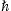= c = 1 can easily be restored. We use the Heaviside–Lorentz system, so that αq = q2/(4πc) ≈ 1.0 × 10−13. The tachyon mass is mt ≈ 2.15 keV/c2, the tachyon–electron mass ratio mt/m ≈ 1/238. These estimates are obtained from hydrogenic Lamb shifts . The tachyonic count rates (tachyons emitted per unit time and unit frequency interval) are nT,L(ωpT,L(ω)/ω.

The radiation densities (1) refer to a single charge with Lorentz factor γ. We average them with electron distributions, power-laws exponentially cut with Boltzmann densities, dρ ∝ E−2−αeE/(kT)d3p, or

where βmc2/(kT). At α = −2, the distribution is thermal. The electronic Lorentz factors range in an interval γ1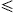γ < ∞. The normalization factors Aα,β(γ1, n1) of densities (2) are determined by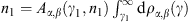, where n1 is the electron count and γ1 the smallest Lorentz factor of the source population.

The averaging is carried out via

with ωmax(γ) defined above. These averages can be reduced to the spectral functions

where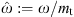and ΔT,L as in (1).

The spectral range of the radiation densities (1) is 0 < ω < ωmax(γ). Inversely, the conditionordefines the minimal electronic Lorentz factor for radiation at this frequency. (stands for the rescaled frequency ω/mt.) That is, an electron in uniform motion can radiate at ω only if its Lorentz factor exceeds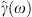. The lower edge of Lorentz factors in the electronic source distribution defines the break frequency, ω1ωmax(γ1), or, which separates the spectrum into a low- and high-frequency band. We have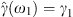, andif ω > ω1. The averaged energy density (3) can thus be assembled as

The superscripts T and L denote the transversal and longitudinal polarization components. If the polarization is not distinguished, we add the polarization components, writingpT+Lαβ for the unpolarized density.

To obtain the low-temperature expansion, β1, of the spectral average (5), we substitute the asymptotic expansion of the incomplete Γ-function  into the spectral function BT,L(ω;γ;α,β),

The expansion parameter is βγ1. The high-temperature expansion, β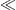1, of BT,L in (4) is based on the ascending series of Γ(−α − 1, βγ),

which requires βγ1. In the opposite limit, βγ1, we may still use the low-temperature expansion (6), even if β1. Singularities occurring at integer α in (7) cancel if ε-expanded.

If γ1 = 1, β1 and α > 1, the maximum ofpT,L(ω; γ1, n1)αβ, cf. (5), is determined by the lead factor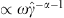in the high-temperature expansion (7). This peak is located atand is followed by power-law decay ∝ωα for ωmt/β and exponential decay starting at about ω ≈  mt/β. The low-temperature expansion (6) applies for ωmt/β. If γ11 (but still βγ11 and α > 1), the peak ofpT,L(ω;γ1n1)αβ is determined by the factor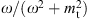in (7) and occurs at ωmax ≈ mt. It is followed by a broken power-law, at firstpT,Lαβ ∝ 1/ω, in the range 1ω/mtγ1, and thenpT,Lαβ ∝ ωα in the interval γ1ω/mt1/β. At ω ≈ mt/β, there is an exponential cutoff according to (6).

The spectral fit is performed with the E2-rescaled flux density E2dNT,L/dE, which relates to the energy densitypT,Lαβ in (5) as

where d is the distance to the pulsar. The preceding scaling relations forpT,Lαβ give a plateau value E2dNT,L/dE ∝ 1 in the range 1E/(mt c2γ1, followed by power-law decay E2dNT,L/dE ∝ E1−α in the band γ1E/(mt c21/β, and subsequent exponential decay.

In the foregoing discussion of the high-temperature asymptotics, we assumed an electron index α > 1. We still have to settle the case α < 1. The spectral peak at ωmax ≈ mt is again determined by the factor. Adjacent is a power-law slopepT,Lα,β ∝ 1/ω in the range 1ω/mt1/β, so that E2dNT,L/dE ∝ 1. At ω ≈  mt/β, there is the cross-over to exponential decay, cf. (6). This holds true for γ1 = 1 as well as γ11, provided βγ11, since the leading order of the high-temperature expansion (7) does not depend on γ for α < 1. If βγ11 despite of β being small, then the low-temperature expansion (6) applies instead of (7).

### 3. Tachyonic cascade spectra and spectral fits

Fig. 1, Fig. 2 and Fig. 3 show the E2-scaled differential number flux, E2dNT+L/dE, as defined in (8), fitted to the data points. As the polarization is not observed, we add the transversal and longitudinal densities, writingpT+LpT+pLfor the energy density and dNT+LdNT + dNL for the number flux. We restore the natural units on the right-hand side of (8), up to now we have used= c = 1, and write E(1) forω(1). The spectral maps are in MeV units, with the differential tachyon flux dNT,L/dE in units of MeV−1s−1cm−2. The normalization factor in (5) is dimensionless,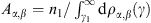, where n1 is the number of radiating electrons with Lorentz factors exceeding γ1, distributed according to density (2). α is the electronic power-law index, and β the exponential cutoff in the electron spectrum, both to be determined from the spectral fit like n1 and γ1. As for the electron count n1, it is convenient to use a rescaled parameter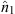for the fit,

which determines the amplitude of the tachyon flux. Here,[keV s] implies the tachyon mass in keV units, that is, we put mtc2 ≈ 2.15 in the spectral function (4). The purpose of this rescaling is to avoid the distance estimate in the actual fitting procedure. Onceis extracted from the spectral fit, we can calculate via (9) the number of radiating electrons n1 constituting the density dρα,β. This, however, implies that all tachyons reaching the detector are properly counted. At γ-ray energies, only a tiny αq/αe-fraction (the ratio of tachyonic and electric fine structure constant, αq/αe ≈ 1.4 × 10−11) of the tachyon flux is actually absorbed, cf.  and Section 4. This requires a rescaling of the electron count n1, so that the actual number of radiating electrons is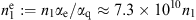. In Table 1, we list the flux amplitudeinferred from the spectral fit, as well as the renormalized electron count. (In the table, we drop the subscript 1 inand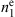.) The density dρα,β is defined by parameters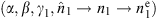obtained from the spectral fit.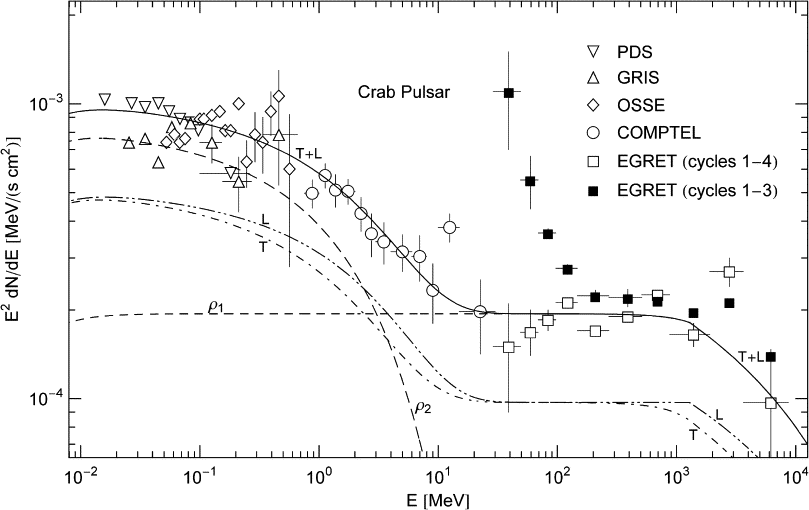Fig. 1. Tachyonic spectral map of the Crab pulsar. PDS, COMPTEL and EGRET (open squares) data points from , GRIS points from , OSSE points from ; also see ,  and  for COMPTEL data on the pulsed Crab emission. The open squares are the final EGRET data covering cycles 1–4, corrected for spark chamber gas aging . For comparison, we also indicate the EGRET flux points based on cycles 1–3, cf. ; the error bars on the last four flux points are suppressed, they are similar to those on the open squares with the same abscissa. In the χ2-fit, only the final EGRET data (open squares) are considered, and the vertical error bar on the second flux point is assumed to hold for the subsequent six points as well. The solid line T + L is the unpolarized differential tachyon flux dNT+L/dE, rescaled with E2 for better visibility, cf. (12). The total flux T + L can be split into transversal (T, dot–dashed) and longitudinal (L, dot–dot–dashed) flux components dNT,L/dE, cf. (13). The cascade spectra labeled ρ1,2 (dashed) are the unpolarized flux densities dNT+L(E,ρi)/dE of two electron populations ρi=1,2, cf. Table 1 and (14). These cascades add up to the total flux T + L, which is the actual spectral fit with parameters (of the electron densities ρi=1,2) listed in Table 1. The MeV plateau terminates in a nearly straight GeV power-law slope. In contrast to the ρ2-cascade, the cutoff temperature of ρ1 is too high to significantly curve the power-law slope in the energy range covered here, cf. Fig. 3 for the wideband view. A spectral break at E ≈ mtγ1 ≈ 1.3 GeV is visible as edge in the longitudinal spectral map, cf. after (8). ρ1 is the cascade producing the MeV plateau and the adjacent GeV power-law slope ∝1/E (α = 1, cf. Table 1). The soft γ-ray band is generated by adding the flux of the ρ2-cascade to the ρ1-plateau. The spectral curvature is caused by the Boltzmann factor in the averaged spectral densities, whose cutoff frequency is Ecut = (mt/m)kT, defined by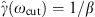according to the low-temperature/high-frequency expansion (6). This implies cutoffs at 88 GeV for the ρ1-cascade and 6.3 MeV for ρ2. The cutoff temperature of the respective electron densities is recorded in Table 1. This figure is a close-up of the γ-wideband in Fig. 3.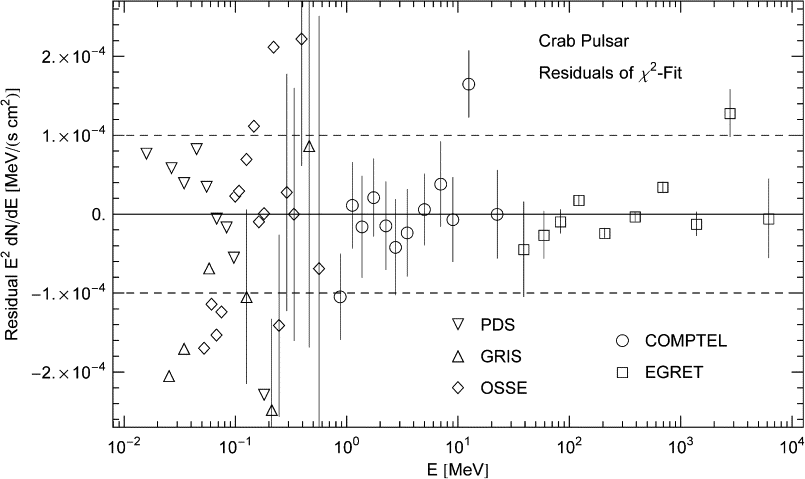Fig. 2. Residuals of the least-squares fit in Fig. 1. The cascade ρ1 in Fig. 1 is based on ten EGRET flux points; the ninth point above the 10−4-mark is discounted in the χ2-fit. This cascade is fitted with χ2 ≈ 3.85 (unreduced) and a confidence level of 57% with 5 dof. The cascade depends nonlinearly on the four fitting parameters, which limits the significance of Gaussian p-values, Γ(dof/2,χ2/2)/Γ( dof/2), apart from the low statistics. For instance, the first seven points of the EGRET plateau amount to a horizontal straight-line fit effectively depending on only one independent fitting parameter, which suggests a much higher confidence level. The ρ2-cascade in Fig. 1 on top of ρ1 is based on the COMPTEL points. The first and last of the indicated 12 COMPTEL points are discarded in the fit, and χ2 is weighted with the indicated error bars. We find χ2 ≈ 1.40 and a confidence level of 97% with 6 dof. This high p-value is mainly due to the larger error bars, as compared to the EGRET fit. The PDS, GRIS and OSSE points are not included in the χ2-fit of the ρ2-cascade, but it is evident from the rather symmetrically spaced residuals that the cascade is a good fit to these dispersed data sets. The residuals (data points minus fitting function (12), (13), (14) and (15) with parameters as in Table 1) have not been normalized.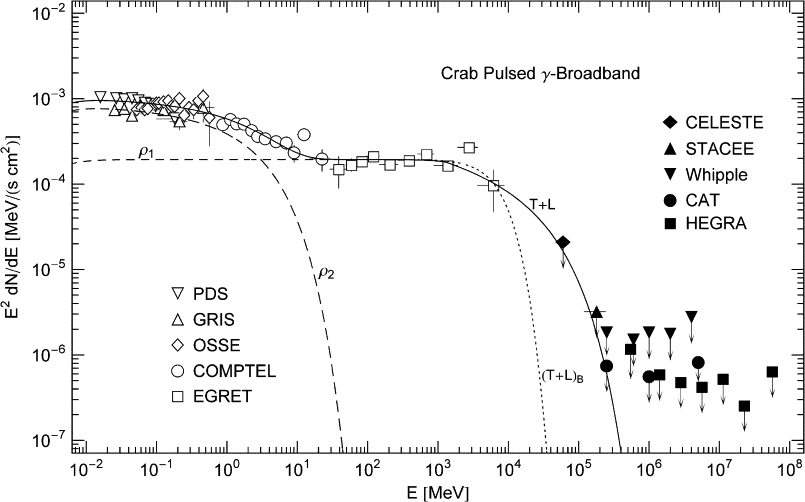Fig. 3. γ-Ray wideband of the Crab pulsar. Data points depicted as open symbols are referenced in Fig. 1. Filled symbols (upper bounds) from CELESTE , STACEE , Whipple , CAT , and HEGRA . The spectral fit T + L (solid line) is done with the rescaled unpolarized tachyon flux E2dNT+L/dE as in Fig. 1. The GeV power-law slope following the spectral break at 1.3 GeV terminates in exponential decay. The GeV-fit hinges on the last EGRET point, the CELESTE and STACEE points, and the first CAT point. The latter three data points are upper limits. Another option for the high-energy fit is the dotted extension (T + L)B of the MeV plateau, fitted to the EGRET points only, the upper bounds are evidently satisfied. This fit is based on a thermal Boltzmann distribution generating the ρ1-cascade, the parameters of the electron density ρ1 in Table 1 are replaced by α = −2, β ≈ 7.17 × 10−7 and γ = 1, andremains unchanged. In this case, there is no power-law interface between the MeV plateau and exponential decay. Presently, there are not enough data points in the GeV band to discriminate between the exponentially cut power-law fit T + L and the thermal fit (T +  L)B.

Table 1.

Electronic source densities ρi generating the tachyonic cascade spectra of the Crab pulsar

α β γ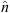ne kT (TeV)
ρ1 1.0 2.39 × 10−8 6.0 × 105 2.1 × 10−2 4.8 × 1048 21
ρ2 0.6 3.31 × 10−4 1 9.0 × 10−2 2.1 × 1049 1.5 × 10−3

Each distribution is defined by parameters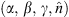, cf. Eq. (2). α is the electronic power-law index, β the cutoff parameter in the Boltzmann factor, and γ the lower edge of Lorentz factors in the source population.is the amplitude of the tachyonic flux density generated by ρi. The electron count ne is inferred fromvia (9) and d ≈ 2 kpc. kT is the electron temperature. The ρ1,2-cascades in Fig. 1 and Fig. 3 are obtained by averaging the superluminal radiation densities (1) with the electron distributions ρ1,2 listed here. The parametersare extracted from the spectral fit.

In the case of a thermal electron distribution (α = −2), we may identify kT [TeV] ≈ 5.11 × 10−7/β. We use this definition of electron temperature also for other electron indices α. kT is the energy at which the exponential decay of the electron density (2) sets in. At high temperature, density (2) is peaked at γpeak ≈ −α/β, provided α < 0 and βα; the peak is followed by exponential decay. If α > 0, the electron density is decreasing monotonically, at first as power-law and exponentially beyond kT. Nearly straight power-law slopes, as seen in the low GeV range of Fig. 1, can only emerge for electron indices α > 1, cf. after (7). The spectral break from the MeV plateau into the power-law slope is determined by the lower edge γ1 of electronic Lorentz factors, cf. after (4). If α < 1, the MeV plateau is followed by exponential decay, without power-law cross-over, as exemplified by the thermal cascade (T + L)B in Fig. 3.

Wideband cascade spectra are obtained by averaging over multiple electron populations,

Similar linear combinations, including pure power-laws, have been used to model γ-ray burst spectra  and  and electron distributions in galaxy clusters . The normalization factors Aαi,βi(γi,ni) of the individual averages in this series are calculated via

where ni is the electron count and γi the smallest Lorentz factor in the population defined by dραi,βi. The electron numbers ni (attached to a power-law index αi, temperature βi = mc2/(kTi), and interval boundary γi) determine the weights in the linear combination (10).

We summarize the flux density plotted in Fig. 1 and Fig. 3. The spectral fit is done for unpolarized radiation, so that we start by summing over the polarization components,

We plot the unpolarized E2-scaled flux density E2dN/dE, as well as the polarization components E2dNT,L/dE. (We occasionally use a superscript T + L to indicate the total flux density, writing dNT+L instead of dN.) The polarization components in (12) are obtained by summing over two cascades, cf. (8) and (10),

The individual cascades used in the least-squares fit described in the caption of Fig. 2 are assembled from (2), (4), (5) and (8). Restoring dimensions, we find their explicit shape as

Each cascade depends on four fitting parameters, αi, βi, γi, ni, whose meaning is explained at the beginning of this section. [The actual spectral fit described in the figure captions is done by at first summing over the polarizations in (14) and by adding the χ2-fits of the unpolarized cascades E2dNT+L(E;αi, βi, γi, ni)/dE. That is, we interchange the summations in (12) and (13) to obtain E2dN/dE.] θ is the Heaviside step function, and we use the shortcut

where we put ΔT = 1 and ΔL = 0, for transversal and longitudinal polarization, respectively.

The total tachyonic flux density E2dN/dE is plotted in Fig. 1 and Fig. 3 (solid line T + L). The individual unpolarized cascades, E2dNT+L(E;αi, βi, γi, ni)/dE, obtained by summing over the polarizations in (14), are labeled ρi=1,2 (dashed curves). They add up to the total tachyonic flux density labeled T + L. The electron densities ρi in Table 1 produce the cascade spectra ρi and are in turn defined by parameters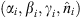obtained from the least-squares fit.

The cutoff kT in the electron energy is listed in Table 1, for each electron population generating a cascade in the γ-ray wideband. In the case of protonic source particles, the cutoff energies have to be multiplied with 1.8 × 103, the proton/electron mass ratio. These cutoffs are to be compared to two spectral breaks in the cosmic-ray spectrum, occurring at 103.5 TeV and 105.8 TeV, dubbed knee and second knee, respectively . A protonic source density generating the ρ1-cascade has a cutoff at about 104.6 TeV, suggesting protons in the pulsar magnetosphere with energies between the first and second knee.

### 4. Conclusion

We have considered superluminal spectral densities generated by freely moving electrons, discussed the spectral averaging with electronic source distributions, and derived the high- and low-temperature expansions of the averaged radiation densities. We demonstrated that the γ-ray flux of the Crab pulsar can be reproduced by tachyonic cascade spectra, and explained the spectral plateau, the power-law slopes, and the spectral curvature.

Here, we have studied superluminal radiation from electrons in uniform motion. Tachyonic synchrotron and cyclotron radiation was investigated in  and . In the zero magnetic field limit, the tachyonic synchrotron densities converge to the spectral densities (1) for uniform motion. Electromagnetic synchrotron radiation vanishes in this limit, of course. A finite bending radius induces modulations in the spectral densities. These oscillations are quite small, just tiny ripples along the spectral slope with increasing amplitude toward the spectral break . If integrated over a thermal or power-law electron distribution as done here, these oscillations are averaged out.

Several electromagnetic radiation mechanisms have been invoked to model the spectra of γ-ray pulsars, most notably curvature radiation due to electric fields followed by pair creation, accompanied by synchrotron radiation and inverse Compton scattering . These mechanisms are quite interlocked, cf. the flow-chart in , and subject to frequent revisions  and . An electromagnetic spectral fit to the pulsed Crab emission, directly comparable to Fig. 1, can be found in Fig. 11 of .

In Section 3, we pointed out that only a fraction αq/αe ≈ 1.4 × 10−11 of the tachyon flux arriving at the detector is actually absorbed, so that the measured flux has to be rescaled with the ratio of electric and tachyonic fine structure constant. This rescaling applies to γ-ray spectra only, to frequencies much higher than the 2 keV tachyon mass, so that the mass-square can be dropped in the tachyonic dispersion relation. At X-ray energies, we have to rescale with the respective cross-section ratio, σe(ω)/σq(ω), where σe is the electromagnetic cross-section and σq its tachyonic counterpart. The detection mechanism for hard and high-energy X-rays is usually ionization, generating scintillations in NaI(Tl) and CsI(Na) crystals of phoswich detectors , or gas scintillations by ionization of Xenon atoms in proportional counters . Tachyonic ionization cross-sections of hydrogen-like ground states, that can be used for X- as well as γ-rays, have been calculated in . At γ-ray energies, photonic and tachyonic dispersion relation coincide, and the cross-section ratio of the detection mechanism (such as ionization , Compton scattering  and pair production , the latter two quantified by Klein-Nishina and Bethe–Heitler cross-section) approaches a constant limit value αe/αq, which amounts to an overall rescaling of the tachyonic flux amplitude. By contrast, at X-ray energies, we would have to rescale the tachyonic flux density with the energy dependent cross-section ratio, which affects the shape of the spectral map. If a grating spectrometer is used, the interference peaks are determined by wavelength. In this case, the observational plots have to be reparametrized by wavelength, and then the tachyonic dispersion relation is substituted to obtain the actual energy-flux relation.

### Acknowledgement

The author acknowledges the support of the Japan Society for the Promotion of Science. The hospitality and stimulating atmosphere of the Centre for Nonlinear Dynamics, Bharathidasan University, Trichy, and the Institute of Mathematical Sciences, Chennai, are likewise gratefully acknowledged.

### References

 J.A. Wheeler and R.P. Feynman, Rev. Mod. Phys. 17 (1945), p. 157.

 R. Tomaschitz, Class. Quantum Grav. 18 (2001), p. 4395.

 R. Tomaschitz, Physica A 320 (2003), p. 329.

 R. Tomaschitz, Eur. Phys. J. B 17 (2000), p. 523.

 W. Magnus, F. Oberhettinger and R.P. Soni, Formulas and Theorems for the Special Functions of Mathematical Physics, Springer, New York (1966).

 R. Tomaschitz, Astropart. Phys. 23 (2005), p. 117.

 L. Kuiper et al., Astron. Astrophys. 378 (2001), p. 918.

 L.M. Bartlett et al., AIP Conf. Proc. 304 (1994), p. 67.

 M.P. Ulmer et al., Astrophys. J. 432 (1994), p. 228.

 R. Much et al., Astron. Astrophys. 299 (1995), p. 435.

 R. Much et al., Astron. Astrophys. Suppl. Ser. 120 (1996), p. 703.

 R.D. van der Meulen et al., Astron. Astrophys. 330 (1998), p. 321.

 R.C. Hartman et al., Astrophys. J. Suppl. 123 (1999), p. 79.

 J.M. Fierro et al., Astrophys. J. 494 (1998), p. 734.

 M. de Naurois et al., Astrophys. J. 566 (2002), p. 343.

 S. Oser et al., Astrophys. J. 547 (2001), p. 949.

 R.W. Lessard et al., Astrophys. J. 531 (2000), p. 942.

 A. Musquère et al., in: D. Kieda, M. Salamon, B. Dingus (Eds.), Proceedings of the 26th International Cosmic Ray Conference, Salt Lake City, 1999, vol. 3, p. 460.

 F. Aharonian et al., Astrophys. J. 614 (2004), p. 897.

 D. Band et al., Astrophys. J. 413 (1993), p. 281.

 N.M. Lloyd and V. Petrosian, Astrophys. J. 511 (1999), p. 550.

 V. Petrosian, Astrophys. J. 557 (2001), p. 560.

 M. Nagano and A.A. Watson, Rev. Mod. Phys. 72 (2000), p. 689.

 R. Tomaschitz, Physica A 335 (2004), p. 577.

 R. Tomaschitz, Eur. Phys. J. C 45 (2006), p. 493.

 K.S. Cheng, C. Ho and M. Ruderman, Astrophys. J. 300 (1986), p. 500.

 C. Ho, Astrophys. J. 342 (1989), p. 396.

 K.S. Cheng, M. Ruderman and L. Zhang, Astrophys. J. 537 (2000), p. 964.

 J. Takata et al., Mon. Not. R. Astron. Soc. 366 (2006), p. 1310.

 F. Frontera et al., Astron. Astrophys. Suppl. Ser. 122 (1997), p. 357.

 G. Manzo et al., Astron. Astrophys. Suppl. Ser. 122 (1997), p. 341.

 R. Tomaschitz, Eur. Phys. J. D 32 (2005), p. 241.

 W.N. Johnson et al., Astrophys. J. Suppl. 86 (1993), p. 693.

 V. Schönfelder et al., Astrophys. J. Suppl. 86 (1993), p. 657.

 D.J. Thompson et al., Astrophys. J. Suppl. 86 (1993), p. 629.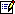Tel.: +81 824 247361; fax: +81 824 240717.